﻿ lattice gauge QCD, extrapolation of hadron pion masses

# Quantum chromodynamics (QCD) uses artificial tricks in hadron masses.

## Unrealistic elementary particles.

### [ W boson (= 80 times proton mass ) is NOT equal to β decay. ]

(Fig.1) W boson in accelerator is completely different from that of β decay.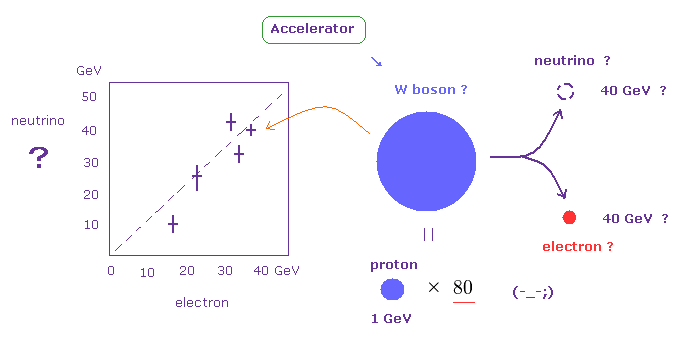First, we refer to unreality of standard model, which is the origin of QCD.
W ( weak ) boson is said to be generated by collisions between some high energy particles in the accelerator.

Unrealistic W boson cannot be observed directrly.
So, by detecting the final product such as electron and neutrino, which has as much as 40 GeV energy, they just estimate the mass of virtual W boson.

The important point is that we cannot detect neutrino at all, as shown on this page, because neutrino can pass through even the earth easily.
They just estimate this unseen neutrino from some loss (= bias ) of the final product's energy.

## Total energy of W boson is NOT conserved in β decay.

(Fig.2) Total energy ( W boson ) is NOT conserved in β decay ? ← unrealistic !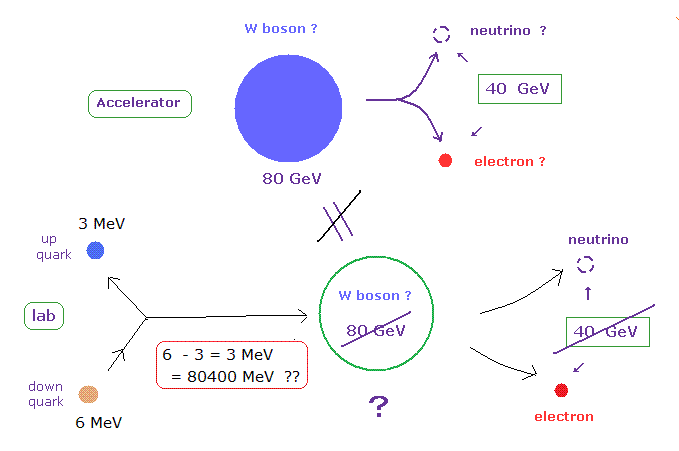The extremely heavy mass (= 80 GeV is 80 times proton's mass ) of this W boson is gotten from data based on the high energy accelerator.
So this W boson in the accelerator is completely different from that of beta decay in the laboratory.

In usual β decay, down quark inside neutron changes into up quark inside proton emitting negative W boson, they insist.
But the energy difference between down and up quarks is only 3 MeV, which is much smaller than the W boson mass (= 80 GeV = 80000 MeV ).

So if W boson in β decay is equal to that of the high energy accelerator, the basic principle of energy conservation is completely violated, which means W boson is a unreal particle.

## Neutral Z boson cannot be distinguished from electromagnetic force.

(Fig.3) Unrealistic Z boson is real ? → It's only electric force ?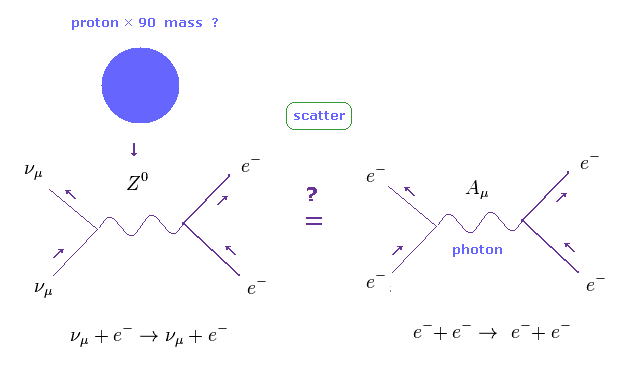They insist the resonant point at about 90 GeV in the accelerator indicates the existence of Z boson.
The important point is that neutral Z boson cannot be distinguished from usual electromagnetic force.
Because the photon (= A ) is also neutral.
So some of Z boson decays in the accelerator may have been just usual Coulomb scattering or something.

## Accelerator cannot collect all scattered energies.

(Fig.4) Z boson and W boson are the same thing ??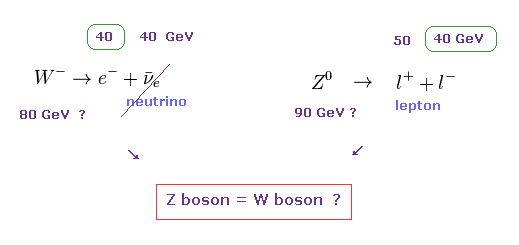90 GeV of Z boson is very close to 81 GeV of W boson.
So each final lepton ( electron etc. ) has about 40 GeV in both Z and W bosons.

Considering unseen neutrino (= a half of W boson's final product ) cannot be detected clearly, it is possible that we just see the same phenomenon in both Z and W boson decays.
( So, it is possible that "virtual" Z and W bosons in the accelerator may be the same thing. )

## Quantum choromodynamics (QCD) is an origin of nuclear mass ?

(Fig.5) Symmetry breaking = quark-gluon aggregation ??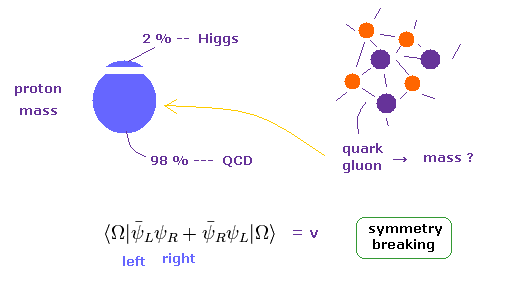It is said that 98 % of nuclear mass is generated by chiral symmetry breaking, and only 2 % of mass is caused by Higgs mechanism.
Then what is chiral symmetry ?

Unfortunately, there are NO concrete physical images in this chiral symmetry (= QCD ), as shown in Fig.5.
( These are only abstract "math" symbols. )

Physically, they insist this QCD mass is caused by various interactions among (sea) quarks and gluons inside a proton.
But of course, they cannot visualize these imaginary state clearly.

## Higgs always breaks a proton to reach and give mass to quarks ?

(Fig.6) Higgs always breaks a proton to reach "up" and "down" quarks ??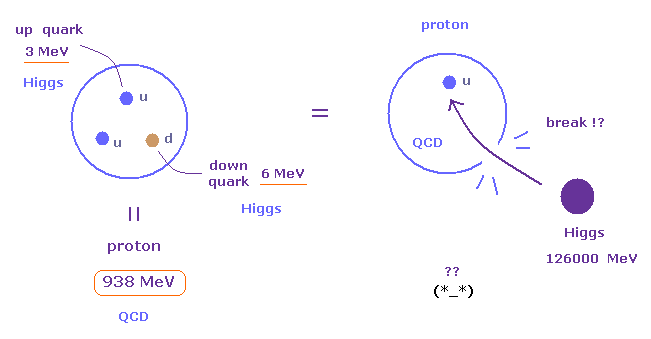Almost all mass of proton is caused by QCD mechanism, and NOT by Higgs.
Only very small "up" and "down" quarks are given their mass from Higgs particle.
This means Higgs particles in the vacuum always break the proton's shell to reach each quark ??

If each proton is "broken" by Higgs, it is completely inconsistent with very stable actual proton.
So the mechanism of acquiring mass by a combination of Higgs and QCD is very unnatural.

On the other hand, they insist Higgs particle can give as much as 174000 MeV mass to a single top quark.
( QCD is NOT involved in this heavy top quark, they insist. Do you think these theories are too good to be true ? )
So, Higgs may be fake ?

## Strong force cannot use usual perturbation methods.

(Fig.7) Strong force cannot predict anything.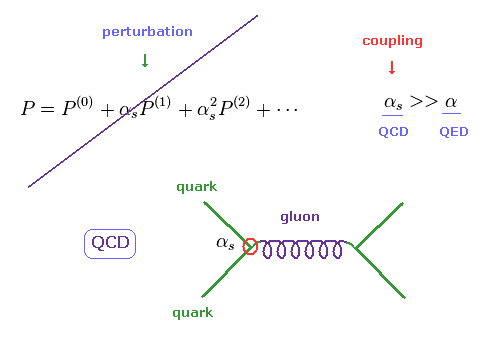The coupling constant of QED (= quantum electrodynamics ) is related to small fine structure constant α (= 1/137 ).
So QED can use perturbation methods to calculate infinite loops caused by virtual particles and photons.

They insist QED can predict the exact values, but as shown on this page, they depend on artificial and wrong math to get anomalous magnetic moment and Lamb shift.

On the other hand, the coupling constant of strong force drastically changes depending on energy.
Under low energy, the coupling constant becomes too strong to calculate the interactions among quarks and gluons.

So we need to depend on some approximate numerical calculation using computers.
This means QCD is NOT an exact solution.

## "Discrete" Lattice QCD is NOT Lorentz invariant.

(Fig.8) Lattice QCD theory is NOT Lorentz invariant.To do numerical calculation, we have to use some lattice structure which has finite spacing (= a ).
Each quark is on each point of this lattice, and gluon is on the line (= link ), which connects two quarks.

Of cource, this finite spacing completely violates Lorentz symmetry of special relativity.
( Seen from horizontal or diagonal directions, this lattice looks different. )

So we have to estimate the values when a = 0, after getting several data of different finite "a".
These manipulations cause various errors in predicting physical values such as hadron masses.

## QCD is based on unrealistic "imaginary" time !

(Fig.9) Imaginary time does NOT describe real phenomena.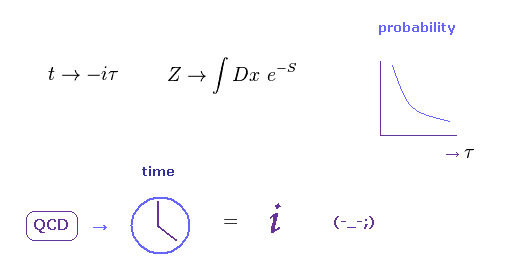The important point is that QCD is based on unrealistic imaginary time (= iτ ) to predict various physical values.
So QCD is basically different from the actual physical world.
For example, experimental proton mass is measured in this real world using "real" ( NOT "imaginary" ) time.

The reason why QCD cannot use the usual time (= t ) is the transition probabitlity (= eiHt ) is oscillating and cannot be determied. ( Imagine some trigonometric functions. )

On the other hand, if we use imaginary time, the probability becomes the exponential function, which does NOT oscillate like "cos" and "sin" functions.
That is to say, various hadron masses based on imaginary time (= QCD ) do NOT mean real mass at all.

## All "superposition" path integral is real ?

(Fig.10) Feynman path integral (= infinite path ) is real ?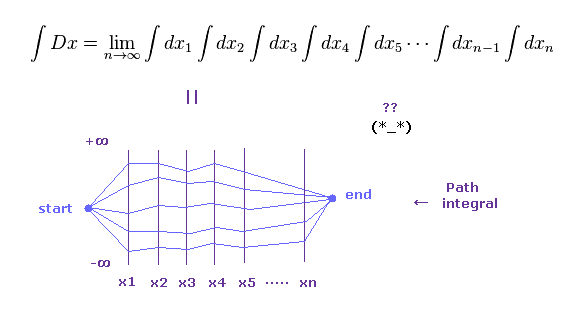The basic concept of QCD is Feynman path integral, in which each particle takes infinite kinds of path at the same time to reach final state.
So these QCD calculations take extremely long time (= about a year ), even if we use a lot of supercomputers.

The idea of path integral is based on unrealistic many-world theory.
From a commonsense point of view, it is impossible that a tiny proton contains infinite kinds of paths of gluons and quarks inside it.

And of course, "imaginary" time is NOT real.
So the current QCD computing is clearly wasting much time, and destroying superior talents of researchers.

## Extremely long QCD calculation is just a waste of time.

(Fig.10') QCD calculation takes extremely much time ( > 1 year ! ) to get some value.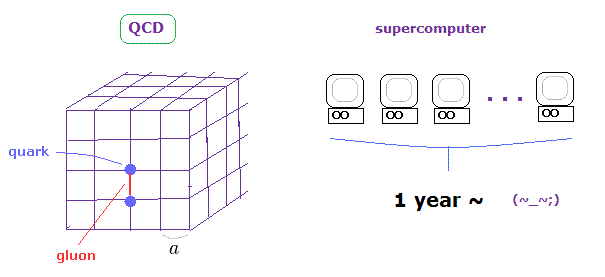Lattice QCD can only give approximate hadron masses.
It gives completely wrong values in nuclear radius, magnetic moment, helicity and quark's momentum.
So QCD has already reached its limit.

## Photon is an origin of "Shut up and calculate !"

### [ Electric "photon" + magnetic "photon" = electromagnetic wave "photon" ?? ]

(Fig.11) Photon has No clear images at all.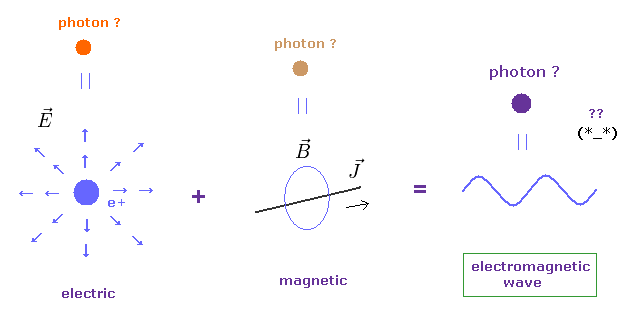As they denied ether, they needed to create ( virtual ) photons to describe electric and magnetic fields.
So, according to the present quantum field theory, each electric and magnetic fields contains photons.

Furthermore, photon is just equal to electromagnetic wave (= light ), they insist.
So a single photon of electromagnetic wave is created by combining electric and magnetic photons ??

This means electromagnetic wave is NOT a single photon ? ( 1 + 1 = "2" photons ? )
So the present quantum theory includes self-contradiction from the beginning.

## "Photon" by quantum electrodynamics (QED) has NO physical entity at all.

(Fig.12) Photon = creation and annihilation operators ??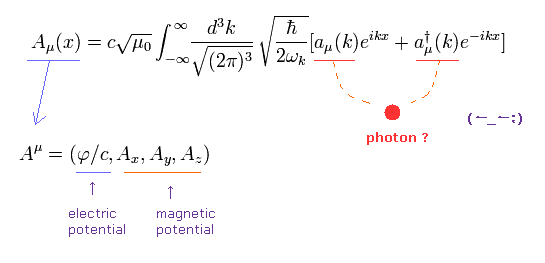Even if you study about quantum field theory, you cannot find what a photon really is.
According to the present QED, the photon is just mathematical creation (= a ) and annihilation (= a ) operators.
These operators are so abstract that they cannot describe the concrete dynamical motions of "photon".

When you want to express a photon using these operators, this single photon needs to be created all over the vacuum.
They cannot designate the specific position of photon due to abstract operators.

Zero component of vector potential (= A0 ) means the electric potential, and 1-3 compotents (= Ai, i = 1, 2, 3 ) mean the magnetic potential.
So "photon" is just a mathematical symbol, NOT a physical thing.

## If electric field consists of "photons", they would run out.

(Fig.13) If electric field is "photon" ..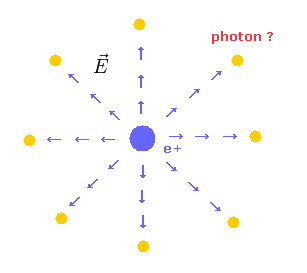If the electric field around some charged particle consists of photons, it means these photons are constantly emitted from the central charge (= e+ ).
This means the stock of photons inside the charged particle would run out, as time goes by ?
This is very strange.

## We cannot advance, unless we clarify what a single photon really is.

(Fig.14)   How big is a photon ? → "Shut up and calculate !"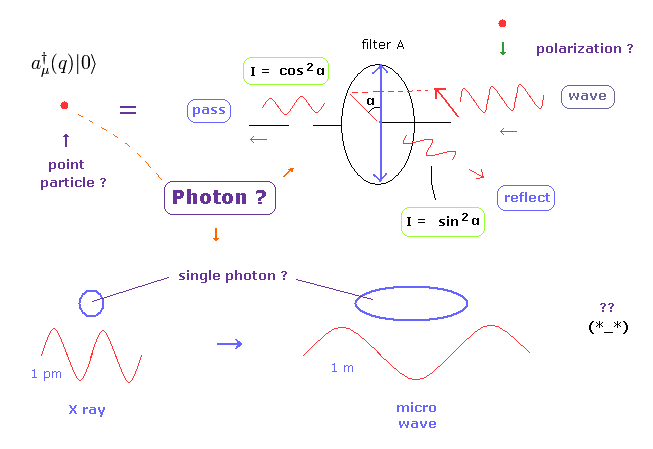Relativistic quantum field theory insists a single photon is a point-like particle.
If so, how that point particle can be polarized as shown on this page ? Impossible.

Wavelengths of X ray and microwave (= both are a single photon ) are about 1 pm and 1 m, which are completely different.
"Heat" itself is one kind of photons, they insist.

## How big is a photon ?   → Shut up !

(Fig.15) "Big Photon" by redshift in Big Bang ? Virtual photon ? Shut up ?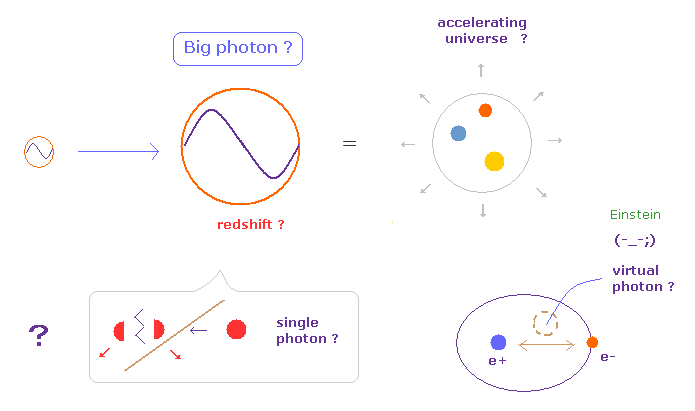As shown on this page, they insist a single photon's wavelength is extremely extended by redshift, while the universe is expanding.
Then we can divide this very extended Big photon into two ?

You may ask the question "How big is a photon ?" to some physicists.
Probably, they just say "Shut up and calculate !", and cannot give clear answer at all.
Furthermore, electromagnetic fields are said to be caused by virtual photons, which violate relativity.

In fact, a strange concept of "photon" plays an important role in blocking and stopping the development of real science.
We have to clarify (= give up ) the single photon, as soon as possible.
They are just classical electromagnetic waves.

## Many unstable new particles are only electrons or protons.

### [ "Muon" is really an elementary particle ? ]

(Fig.16) Muon → electron + neutrino   ← elementary particle ??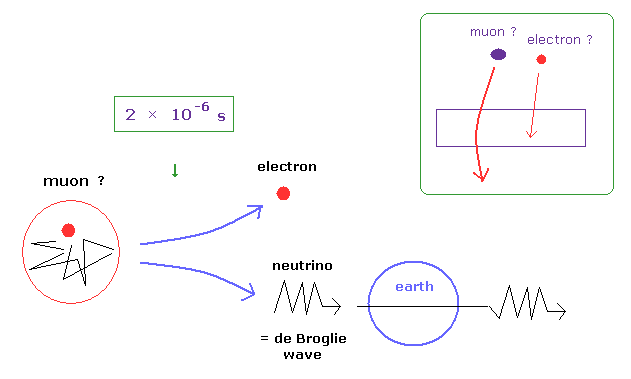Muon was found in 1936 in the cloud chamber.
Though this muon ( lifetime = 2 × 10-6 sec ) easily decays into an electron and neutrino, we can say muon is an elementary particle ??

As muon included in cosmic rays can pass through materials more often than electron, they concluded the muon has about 200 times heavier than an electron.

It is natural that we think very unstable muon is an electron or proton with high energies.
( Almost all of cosmic rays detected on the earth are muons, so it indicates they are common particles. )
Elementary particles must be stable, because they are "elementary".

## Lorentz magnetic force just gives particle's momentum, NOT its mass.

(Fig.17) We cannot know mass and velocity independently.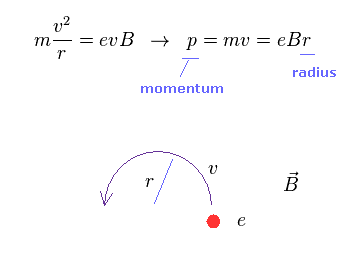Of course, in 1936, there were NO TOF (= time of flight ) detector, which can measure the time that it takes for an object to travel a distance through a medium.
So they just estimate muon's mass only from its track pattern under some magnetic field B.

As shown in Fig.17, if we suppose Lorentz force (= evB ) is equal to centrifugal force, we can know the momentum (= p ) of the moving particle.

The important point is that we cannot know the mass and velocity of the particle only from the cloud chamber.
Then why they could conclude new particle muon has about 200 times heavier than electron ?

## Energy loss inside cloud chamber is doubtful

(Fig.18) Energy loss rate of the moving particle ?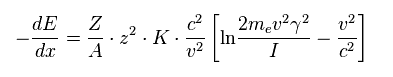They estimate the mass of each particle using the relation of Fig.18.
Fig.18 is the energy loss rate of the particle moving through molecules inside the cloud chamber.
When the velocity v is much slower than the light speed c,

(Fig.19)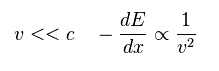From Fig.19, as the velocity (= v ) is slower, the particle tends to lose more energy (= E ), they insist.
So if you believe Fig.18 is right, you can know each particle's velocity only from the track's length.

But of course, there are NO evidence that the relation of Fig.18 is always valid even in the high speed particles.
( Muon inside cosmic rays are as fast as light speed. )

Some physicists at that time insisted the relation of Fig.18 breaks down in the high energy particle, which means the muon is just an electron or a proton with high energy. See also this page.

## Time of Flight (= TOF ) cannot discriminate high speed particles.

(Fig.20) TOF cannot always give correct velocity.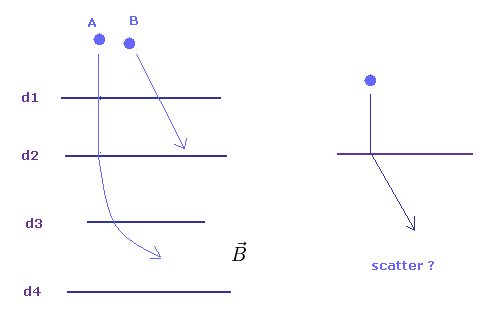Now, the time of flight (= TOF ) detectors are used in discriminating mainly antiparticles such as antiprotons and positrons from electron and protons.
The important point is that TOF has the maximum speed, it can measure, due to the transmitting time of signals.
So, TOF cannot measure the high speed particles in the cosmic rays and accelerator, at all.

Even in low speed particles, they cannot always give correct velocities.
To measure the velocity of some particle, we have to know some exact positions of moving particle at different times.

Coulomb scattering can change particle's velocity and moving direction at each detector (= d1 - d4 ).
So we have to consider unexpected scattering effect to know the precise velocity, which causes various errors.

Furthermore, the number of antiparticles are always much smaller than electrons and protons, so the existence of antiparticle is very vague and doubtful. ( See also this page. )

(Fig.21) Pions and muons are real ?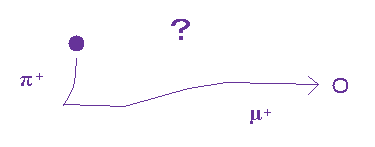The same thing can be said about very unstable pions (= π ).
They just estimate each particle's mass only from the track ( length ) in the cloud chamber.
So these very unstable new particles may be just an electron or a proton ( or other ions )

Fig.21 just shows some scattering in the cloud chamber.
We should NOT increase the number of particle's kinds so easily.
To get true unification theory, the number of elementary particles must be decreased.

## Fractioanl charge = quark was really found ?

### [ Deep inelastic scattering of neutrino really showed quarks ? ]

(Fig.22) 1/3 quarks really exist ?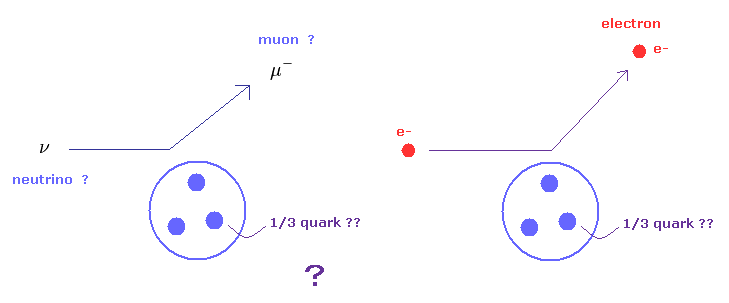Fractional charges such as +2/3e and -1/3e of quarks have NOT been found in experiments untill now.
And according to the standard model, each quark can NEVER be isolated due to strong force.
Though the existence of quark is very doubtful, why they insist quark is real ?
The experimental proof of quarks is based on deep inelastic scattering of neutrino against nucleus.

## Infinite "sea" quarks inside nucleus ?   ← speculation.

But in fact, these scattering experiment completely depends on imaginary sea quarks inside nucleus.
For example, a proton contains two "up" quarks and one "down" quarks.

But the standard model insists a proton contains much more quarks such as "strange" quarks, and antiquarks ....
These virtual quarks are called "sea quarks".
So this theory is a very artificial and ad-hoc one.

(Fig.23) Each quark is NOT 1/3 of proton.   Electron scattering against nucleus.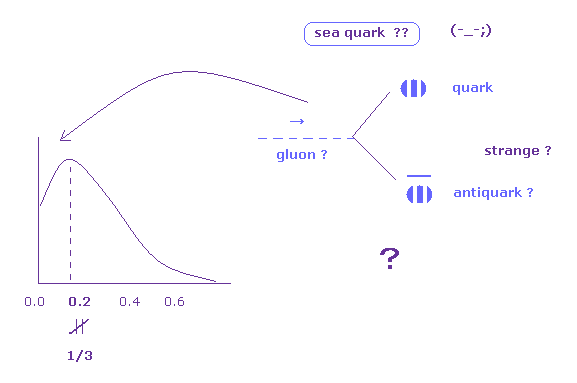As shown in Fig.23 ( see also this page ), the electron's scattering experiments show each quark's energy ( momentum ) is less than 1/3 of the whole proton.
So these scattering experiments cannot show -1/3e or 2/3e fractional charges at all.

They introduced very artificial "sea quarks" inside proton to solve this problem.
"Sea quark" means gluons inside proton generate new pairs of quark and antiquarks, which have smaller momentum.

And due to these sea quarks, each quark's momentum becomes less than 1/3.
But of course, this sea quark can NOT be observed directly. These are only speculations and unrealistic.

## Muon scattering from nucleus.

(Fig.24) Muon scattering from proton or neutron.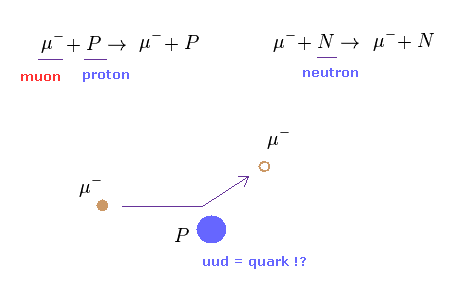They also investigated how often muons (= μ ) is scatterd by a proton or nucleus.
These muons are scatterd by quark's charge inside nucleus, which means Coulomb scattering.

As shown on this page, in classical Rutherford Coulomb scattering, cross section is in proportion to each charge (= sum of a square of each charge ).
A proton contains two "up" quarks and one "down" quark, but they use very ad-hoc assumption like Fig.25.

## Artificial definition = infinite "sea" quarks inside a proton.

(Fig.25) Protons contains all these quarks ??   ↓ sea quarks.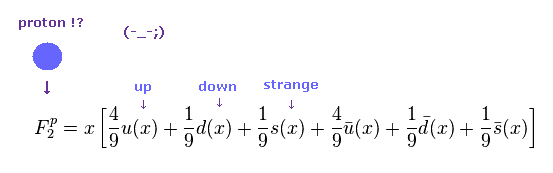Fig.25 is the structual ( = form ) factor of a single proton, which describes proton's internal structure.
Surprisingly, they suppose a proton contains six kinds of quarks such as "up", "down", "strange", and their antiparticles.
( These are virtual "sea" quarks. )
So the assumptions of these scattering experiments rely on wrong assumptions from the beginning.

For example, the charge of "up" quark is +2/3e, so a square of 2/3 is "4/9".
And the "down" quark has -1/3e, so a square of it is "1/9".

(Fig.26) Neutron's structual factor ??   This is strange.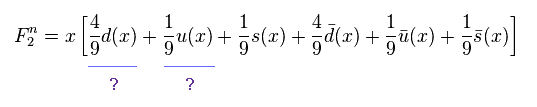Strange to say, they suppose neutron's structual factor as shown in Fig.26.
Though "down" quarks has -1/3e charge, a square of it becomes 4/9 instead of 1/9.
Their assumptions are based on some unrealistic concept of "symmetry".

(Fig.27) Average structual factor of nuclei.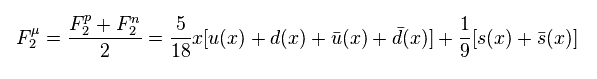From Eq.25 and Eq.26, the average structual factor of nuclei becomes Fig.27.

## Scattering of "ghost" neutrino is very doubtful.

(Fig.28) Neutrino scattering.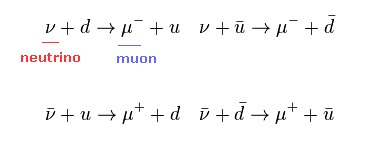The most important experiment is deep inelastic scattering using neutrino.
Of course, almost no neutrinos can be detected. They measure muon as a result of weak interaction.

So this scattering using neutrino is completely different from muon (or electron ) scattering.
Because in Coulomb scattering, almost all scatterd particles can be detected, which is different from "unseen" neutrino.
But they use neutrino scattering data to compare with muon scattering. This is strange.

(Fig.29) Structurel factor by neutrino ?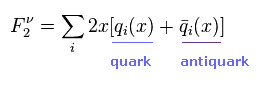Using virtual sea quarks, they suppose the structual factor of nucleus as shown in Fig.29.
Weak force is not related to each charge, so the coefficients such as "4/9" and "1/9" are not included in Fig.29.

(Fig.30) Proton's and neutron's structurel factors by neutrino ?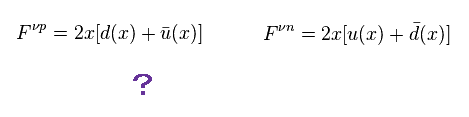Strange to say, they suppose the structual factors of proton and neutron, as shown in Fig.30.
Of course, proton needs to contain two "up" quarks and one "down" quarks, so Fig.30 is wrong.

(Fig.31) Average structurel factor of nuclei ?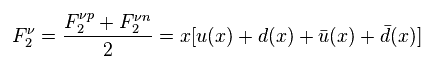From Fig.28 and Fig.30, the average structual factor becomes Fig.31.
When we suppose "strange" quark (= s ) is almost zero, the ratio of Fig.27 (= muon scattering ) to Fig.31 (= neutrino ) becomes

## Standard model prediction by neutrino scattering is doubtful.

(Fig.32) Ratio of muon to neutrino scatterings ?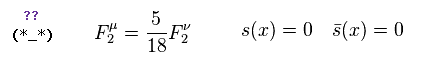This ratio of "5/18" is actually observed in experiments, they insist.
This is the most important experimental proof of fractional charge.
But as shown in this section, these assumptions completely depend on unrealistic sea quarks.

If we suppose proton consists of two "up" quarks and one "down" quark ( neutron = 2 × "down" + one "up" quarks ), the real structural factors become

(Fig.33)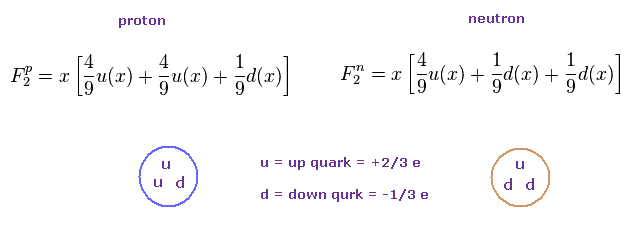So the average structual factor of nucleus is

(Fig.34)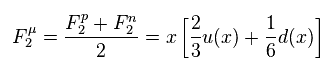Fig.34 is completely different from Fig.27.
So these scattering experiments are based on wrong assumptions.

Furthermore they used "unseen" neutrino for comparison to muon.
But almost all neutrino pass through nuclei without generating weak interactions.
The probability that neutrino interacts with nuclei is quite random, and cannot be known.
So the ratio based on neutrino is very doubtful.

## Lattice QCD depends on artificial methods.

### [ Quantum chromodynamics (= QCD ) is a non-perturbative method. ]

(Fig.35)The coupling constant αs of strong force (= QCD ) is too strong to be applied to usual perturbation methods.
So, all we can do is to do some approximate numerical calculation.
In short, QCD has NO power to predict actual physical values, unless we use some artificial definition and algorithm.

## Discrete lattice is NOT Lorentz symmetry.

(Fig.36) Lattice gauge violates Lorentz symmetry.To do numerical calculation, we have to adopt lattice structure, which have some finite spacing (= a ).
In this lattice, fermions such as quarks are expressed as a point, and gluons are expressed as "link" connecting two fermions.

Of course, as this lattice gauge theory violates Lorentz symmetry of relativity, we have to extrapolate various values to continuum limit ( a → 0 ).
In this process, various errors happen

## Masses of "up", "down" quarks are free parameters of QCD.

(Fig.37) How quark's mass can be determined ?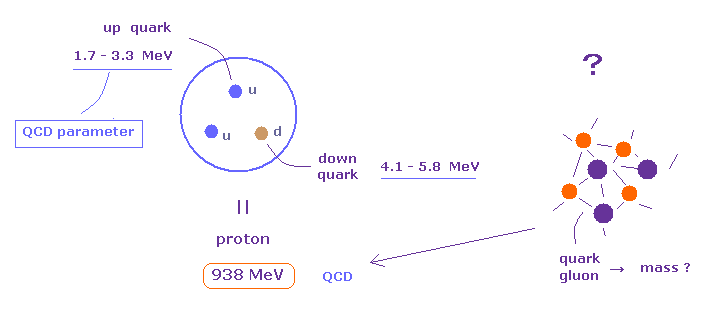In fact, masses of light quarks such as "up", "down" and "strange" quarks cannot be measured in actual experiments.
Then how these quark's masses were determined.

These light quark's masses are free parameters, which we can freely manipulate, in QCD.
To determine these quark's masses, we use some experimental values of three hadron ( meson ) masses.

But as shown in Fig.37, these quark's masses cannot be determined as a single value.
( In "up" quark, the range is 1.7 - 3.3 MeV, its mass can be two times heavier. ).
These results indicate QCD numerical calculation causes various errors.

## Lattice QCD cannot use "real" time.

(Fig.38)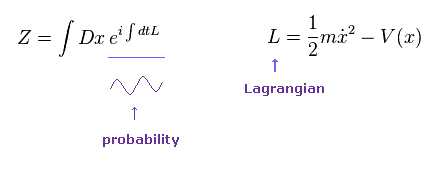As you know, the probability amplitude changes like eiHt in usual quantum theory.
This means the probability is oscillating obeying trigonometric functions.
In this uncertain probability, they cannot get hadron masses at all.

In Fig.38, Z is a partition function, and L is Lagrangian in classical mechanics.

## "Imaginary" time is illusion.

(Fig.39) "Imaginary" time is real ?So we have to change "real" time into "imaginary" time by Wick rotation.
Of course, "imaginary" time is unrealistic, so calculated values by QCD is unrealistic, too.

As shown in Fig.39, if you use imaginary time, the probability amplitude becomes an exponential function, which doesn't oscillate. ( = Euclidean spacetime. )
This transformation is only for calculation, and does NOT show reality at all.

## Infinite path integral is impractical, and a waste of time.

(Fig.40) Path integral = many worlds are real ?Suprisingly, they believe many worlds, so they try to compute all (= infinite ) paths in QCD.
Of course, we cannot calculate infinite paths in lattice QCD.

You need to choose some of paths, which means the results by approximate lattice QCD are intentional things, and NOT true values.

## The form of Lagrangian in QCD can be freely chosen.

(Fig.41) Action of QCD.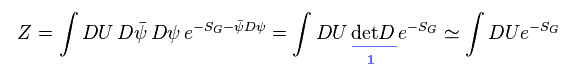Partition function Z of QCD can be expressed like Fig.41.
In Fig.41, the action of SG consists only of gluons.

ψDψ part means Dirac fermions.
As I explain later, these actions (= Lagrangian ) can be freely changed to fit experimental results.
So these parts are, what we call, free parameters.

(Fig.42) Complicated path integral.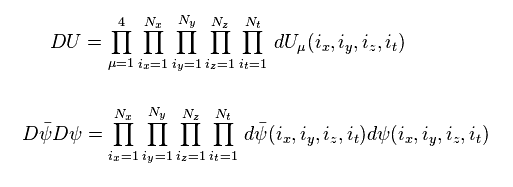As I said, we need to consider all possible paths.
So this many-world like path integral is very unrealistic.
But they believe this strange concept, and are wasting much time for this computing.

## Calculation of path integral is based on "arbitrary" algorithm.

(Fig.43) Probability of gluons.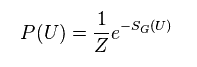It is impossible to compute all paths.
So in lattice QCD, we have to artificially choose some paths in gluon fields.
( There are various methods such as "hybrid Monte Carlo". )

The probability of each gluon path is given like Fig.43.
( This SG can be freely changed under some several conditions. )

## Wilson loop = gluon's path ?

(Fig.44) Wilson loop.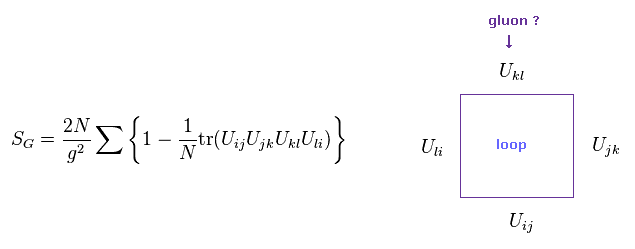Lattice QCD needs to obey gauge symmetry.
So each gluon path needs to form some loop, like Fig.44.
If a loop is formed, they don't change under gauge transformation, as shown on this page.

## Fermion's Lagrangian is "free" parameter.

(Fig.45) Fermion part.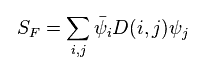To get various hadron masses, we need to use quark fermions.
The action S of fermions is Fig.45.

This action can be freely changed, so there are many types of this action.
Of course, if this action changes, the calculated results change, too.
We can choose some proper action to be close to experimental results.

## Action of QCD can be freely changed to fit experimental results.

(Fig.46) Wilson fermion doesn't satisfy chiral symmetry.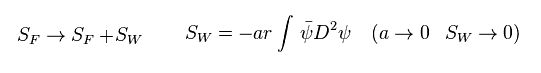In fact, the action S in this lattice theory can be freely changed, which is different from usual quantum field theory.
As shown in Fig.46, if arbitrary actions have the coefficient "a", this action becomes zero in continuum limit ( a → 0 ).

It is known that fermion's action causes serious problems such as "doubler".
To avoid this doubler problem, they artificially add term of Fig.46 to the original Dirac fermion.

This is called "Wilson fermion".
But this Wilson fermion doesn't satisfy chiral symmetry, even when quark mass is zero.
( This is another serious problem. )

## One of them is Wilson fermion.

(Fig.47) Wilson fermion.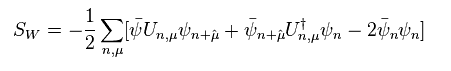Using these actions, we get some gluon gauge configurations and quark's propagators.
As I said, lattice theory is not perturbation, so numerical calculation can give various different patterns in these propagators and gluon's paths.

So depending on these configurations, the calculated results change.

## Lattice QCD takes a great deal of time ( about a year ! ).

It takes extremely much time (= about a year ) in each QCD calculation, even if we use a lot of supercomputers at the same time.
So actually it is impossible to try various patterns.

(Fig.47')   QCD calculation takes extremely much time ( > 1 year ) !## Estimation of light hadron mass.

(Fig.48) Propagator of π meson.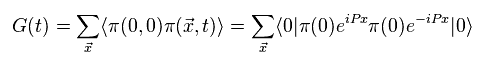Using above quark and gluon's actions, we get propagator of π meson.
In fact, there are various different forms of these hadron's propagators.
So again, we can choose some good form to fit experimental mass.

(Fig.48) π meson mass using imaginary time is real ?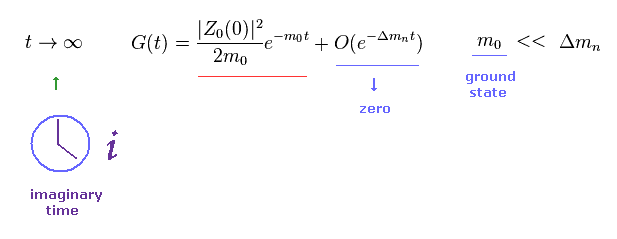The ground state energy (= mass, m0 ) is smaller than any other excited ones.
So as imaginary time t is close to infinity, only the π meson's mass of ground state (= red line ) is left, they insist.

(Fig.49) Effective mass of π meson.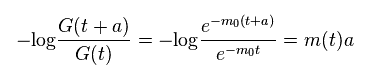When the time is big enough, we can get the relation of Fig.49 from the first term of Fig. 48.

## QCD calculation gives large "error".

(Fig.50) Effective mass of π meson ?   t = free parameter ?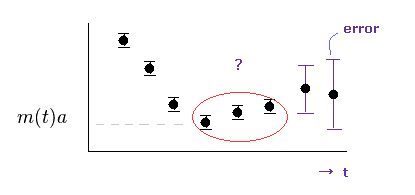To get stable values of π meson, we need to make the imaginary time (= t ) big enough.
But as shown in Fig.50, as this time t becomes bigger, the error becomes bigger, too.
So we pick up the proper values (= red circle ) as π meson mass.
This means this range of time t is also a free parameter, which we can choose.

## We cannot get actual π meson's mass directly by QCD.

(Fig.51) Fitting parameters.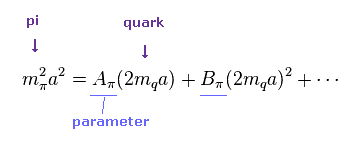The important point is that we cannot get true π meson's mass (= 140 MeV ) directly, using QCD.
Because, to get light mass of 140 MeV, it takes unrealistically long time to compute.
So from several different masses (= more than 200 MeV ), they just estimate π meson's mass.

## Extrapolation of mass = "Rough" estimation.

This technique is called "extrapolation".
Of course, we cannot get all values due to the limitation of calculating time.
Based on several mass points, they try to fit them to experimental values.

In lattice QCD, they try to predict all hadron masses only from (light) quark masses.
Fig.51 shows the relation between "up" ( or "down" ) quark's mass and pion's mass.
The coefficient "A" and "B" .. are fitting (= free ) parameters.

## Fitting functions are "arbitrary".

(Fig.52)The important point is that these fitting functions (= relations between quark's and hadron masses ) are unknown.
So we can freely choose fitting functions.
( In Fig.51, it is a polynominal, and in Fig.52, it is a logarithmic function. )

## Fitting funtions of nuclear mass.

(Fig.53)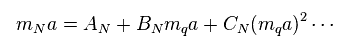In the same way, we choose some functions to express the relation between nuclear and quark's masses.
The coefficients of "A", "B", "C" .. are arbitrary values (= free parameters ).

## Lattice size effect = Error.

(Fig.54)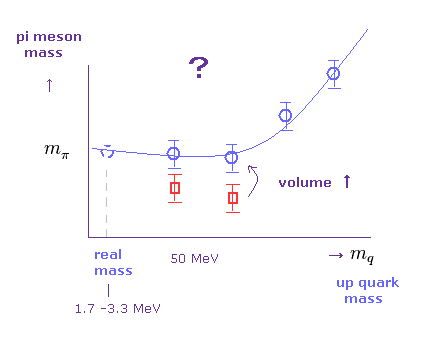We cannot get true pion's mass using true light quark's mass directly in QCD.
Using ( "up" or "down" ) quark's masses, which are much heavier than actual values, they estimate real π meson's mass, as shown in Fig.54.
For example, using 50, 150, 200 MeV "up" quark's masses, they estimate various values.
( Real "up" quark's mass is only 1.7 - 3.3 MeV. )

Of course, the size (= volume ) of the lattice we choose influences the calculated results.
So we can choose proper size to fit experimental values.
( This means QCD is NOT "ab-initio" at all. )

## Extrapolation to continuum limit causes great errors.

(Fig.55)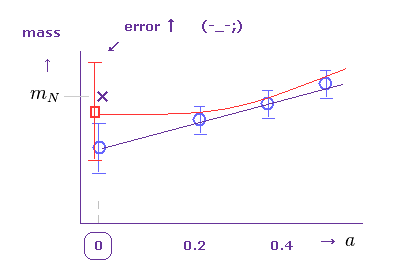We cannot get true values at the zero spacing ( a = 0 ) directly.
So, for example, they estimate physical values based on several other points ( a = 0.2, 0.4 .. ).
This is called "extrapolation to continuum limit", which is the cause of various errors.

By adjusting some fitting parameters, they can choose appropriate physical values.
Again, QCD is NOT ab-initio method.
To reduce these errors, they try to change the actions (= S ) and algorithms, which can be arbitrary.
So, actions and algorithms ( which determine paths ) are also free parameters.

## "Fitting" function can be arbitrary. = free parameter.

(Fig.56)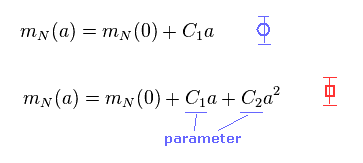In Fig.55, when you choose different fitting functions like Fig.56, the estimated mass of nucleus ( at a = 0 ) is changed.

As a result, the lattice QCD can NOT predict exact values.
They just try to fit calculated values to real values by adjusting fitting parameters and actions.
First, "imaginary" time is NOT real.

## What is chiral symmetry breaking ? They are "math", NOT physics.

(Fig. 57) Chiral symmetry breaking causes 98 % of nuclear mass.In this section, we show the concept of chiral symmetry is very similar to Higgs mechanism , and they are mathematical concepts without physical images at all.

98 % of nuclear mass originates in this chiral symmetry breaking, and only 2 % of nuclear mass originates in Higgs mechanism.

(Eq.1) Right handed and left handed wavefunctions.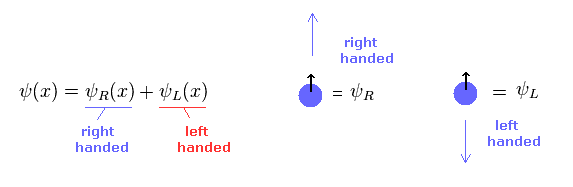Dirac fermion's wavefunction (= ψ ) can be divided into right-handed and left-handed wavefunctions.
"Right handed" means the directions of spin and momentum are the same.
"Left handed" means these directions are opposite to each other.

(Eq.2)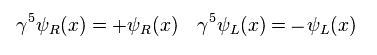where γ5 matrix satisfies
(Eq.3)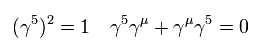Using Eq.2, we can pick up only right-handed ( or left-handed ) wavefunction like
(Eq.4)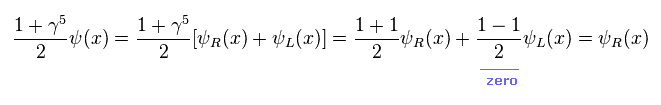As a result,
(Eq.5)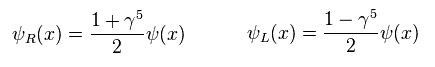As shown on this page, Lagrangian of Dirac field (= fermions such as electrons and quarks ) is
(Eq.6)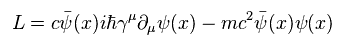where mass term (= second term ) of Eq.6 is
(Eq.7)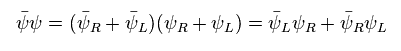Here we explain the reason of Eq.7 result.
In Eq.7, following functions are
(Eq.8)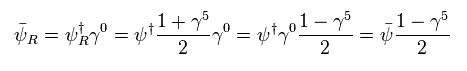and
(Eq.9)From Eq.8 and Eq.9, combinations of "RR" and "LL" wavefunctions become zero.
(Eq.10)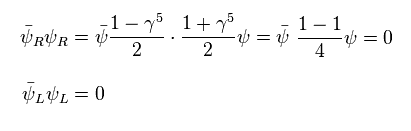So, we can get Eq.7.

On the other hand, the kinetic term (= first term ) of Eq.6 becomes
(Eq.11)Here we use the following relation,
(Eq.12)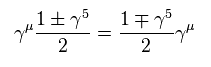Using Eq.8, Eq.9 and Eq.12, we get
(Eq.13)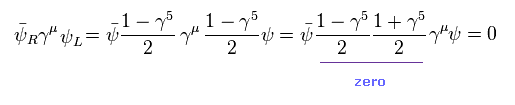We can get Eq.11.

Here we consider the following phase ( chiral ) transformation,
(Eq.14)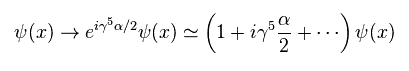Using Eq.2, Eq.3 and Eq.14, right ( or left ) - handed wavefunctions transform like
(Eq.15)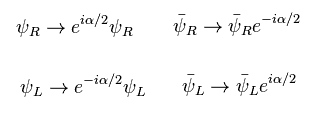As shown in Eq.7, mass term consists of a pair of right and left functions.
So this mass term changes under the phase transformation of Eq.15,
(Eq.16)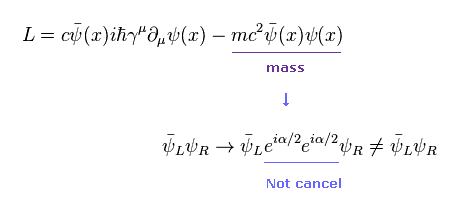So, if Lagrangian of quark contains mass term, it is NOT invariant under the transformation of Eq.15.
This is called "chiral symmetry breaking", which is the origin of nuclear mass, they insist.

(Eq.17)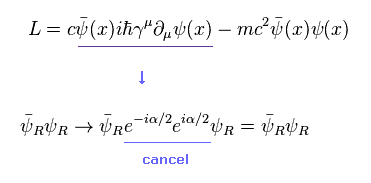On the other hand, kinetic term of Lagrangian is invariant under this transformation.
As shown in this section, the idea of chiral symmetry breaking is very abstract math symbols, and has NO physical reality at all.

### [ Symmetry breaking by Higgs boson. ]

(Eq.18)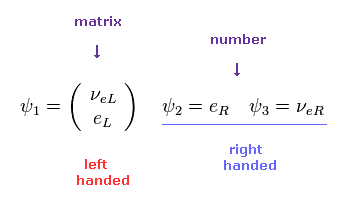As shown on this page, they define only left-handed fermions are "matrix", and right-handed fermitons are "number".
As mass term mixes left-handed and right-handed functions, we cannot find the common phase transformation in mass term.

(Eq.19)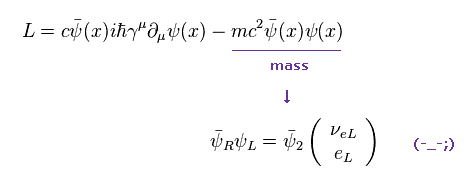Because one is "matrix", and another is "number".
So to satisfy gauge symmetry (= invariance under phase transformation ), this mass term needs to be eliminated, they insist.
This is the reason why they need Higgs boson.
Do you understand this very abstract reason well ?2013/12/3 updated. Feel free to link to this site.